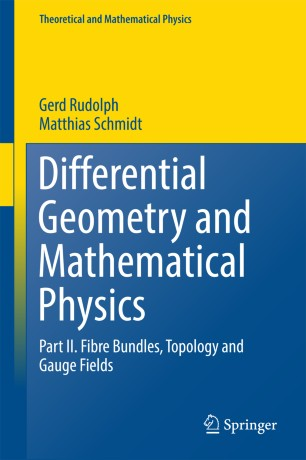# Differential Geometry and Mathematical Physics

## Part II. Fibre Bundles, Topology and Gauge Fields

• Gerd Rudolph
• Matthias SchmidtBook

Part of the Theoretical and Mathematical Physics book series (TMP)

1. Front Matter
Pages i-xvi
2. Gerd Rudolph, Matthias Schmidt
Pages 1-92
3. Gerd Rudolph, Matthias Schmidt
Pages 93-188
4. Gerd Rudolph, Matthias Schmidt
Pages 189-255
5. Gerd Rudolph, Matthias Schmidt
Pages 257-352
6. Gerd Rudolph, Matthias Schmidt
Pages 353-460
7. Gerd Rudolph, Matthias Schmidt
Pages 461-543
8. Gerd Rudolph, Matthias Schmidt
Pages 545-633
9. Gerd Rudolph, Matthias Schmidt
Pages 635-692
10. Gerd Rudolph, Matthias Schmidt
Pages 693-757
11. Back Matter
Pages 759-830

### Introduction

The book is devoted to the study of the geometrical and topological structure of gauge theories. It consists of the following three building blocks:
- Geometry and topology of fibre bundles,
- Clifford algebras, spin structures and Dirac operators,
- Gauge theory.
Written in the style of a mathematical textbook, it combines a comprehensive presentation of the mathematical foundations with a discussion of a variety of advanced topics in gauge theory.
The first building block includes a number of specific topics, like invariant connections, universal connections, H-structures and the Postnikov approximation of classifying spaces.
Given the great importance of Dirac operators in gauge theory, a complete proof of the Atiyah-Singer Index Theorem is presented. The gauge theory part contains the study of Yang-Mills equations (including the theory of instantons and the classical stability analysis), the discussion of various models with matter fields (including magnetic monopoles, the Seiberg-Witten model and dimensional reduction) and the investigation of the structure of the gauge orbit space. The final chapter is devoted to elements of quantum gauge theory including the discussion of the Gribov problem, anomalies and the implementation of the non-generic gauge orbit strata in the framework of Hamiltonian lattice gauge theory.
The book is addressed both to physicists and mathematicians. It is intended to be accessible to students starting from a graduate level.

### Keywords

Dirac operators Linear connections Riemannian geometry Homotopy theory Cohomology theory Whitney sum formula Weil homomorphism Postnikov tower Clifford algebras Spinor groups Spin structures Hodge Theorem Weitzenboeck fromulae Gauge orbit space Gribov problem Index Theorem Yang-Mills equation Seiberg-Witten model Principal bundles Quantum gauge theory

#### Authors and affiliations

• Gerd Rudolph
• 1
• Matthias Schmidt
• 2
1. 1.Institute for Theoretical PhysicsUniversity of LeipzigLeipzigGermany
2. 2.Institute for Theoretical PhysicsUniversity of LeipzigLeipzigGermany

### Bibliographic information

Industry Sectors
Energy, Utilities & Environment